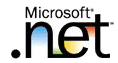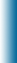Welcome to Mike95.com
 Home Features C++ Library Source Code: COM / ASP Tutorials: Sample Work Internet Based Visual C++ / MFC Java Personal
C++ Templates
Function Templates

To perform identical operations for each type of data compactly and conveniently, use function templates. You can write a single function template definition. Based on the argument types provided in calls to the function, the compiler automatically instantiates separate object code functions to handle each type of call appropriately. The STL algorithms are implemented as function templates.

## Implementing Template Functions

Function templates are implemented like regular functions, except they are prefixed with the keyword template. Here is a sample with a function template.

```#include <iostream>
using namespace std ;
//max returns the maximum of the two elements
template <class T>
T max(T a, T b)
{
return a > b ? a : b ;
}
```

## Using Template Functions

Using function templates is very easy: just use them like regular functions. When the compiler sees an instantiation of the function template, for example: the call max(10, 15) in function main, the compiler generates a function max(int, int). Similarly the compiler generates definitions for max(char, char) and max(float, float) in this case.

```#include <iostream>
using namespace std ;
//max returns the maximum of the two elements
template <class T>
T max(T a, T b)
{
return a > b ? a : b ;
}
void main()
{
cout << "max(10, 15) = " << max(10, 15) << endl ;
cout << "max('k', 's') = " << max('k', 's') << endl ;
cout << "max(10.1, 15.2) = "
<< max(10.1, 15.2) << endl ;
}
```

### Program Output

```max(10, 15) = 15
max('k', 's') = s
max(10.1, 15.2) = 15.2
```
 (c)2023 Mike95.com / Site Disclaimer